# PS Repeating Sequence

Output periodic piecewise linear signal

•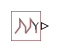Libraries:
Simscape / Foundation Library / Physical Signals / Sources

## Description

The PS Repeating Sequence block outputs a periodic piecewise linear signal, `y`. You can optionally specify an initial signal value and an initial time offset. The repeating sequence consists of a number of linear segments, connected to each other. The number of segments must be no greater than 100. You specify how to connect the segments by choosing a signal type. For the same set of block parameter values, the resulting output signal will be different depending on the signal type:

• `Discontinuous` — Each linear segment in the repeating sequence is defined by its duration, start value, and end value. If the end value of a segment is not the same as the start value of the next segment, they are connected by a vertical line.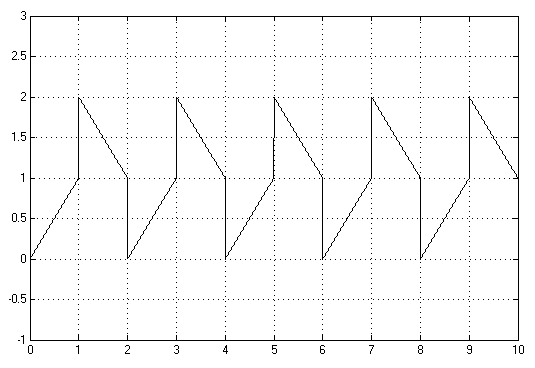• `Continuous` — Each linear segment in the repeating sequence is defined by its duration and start value. The end value of a segment is the same as the start value of the next segment.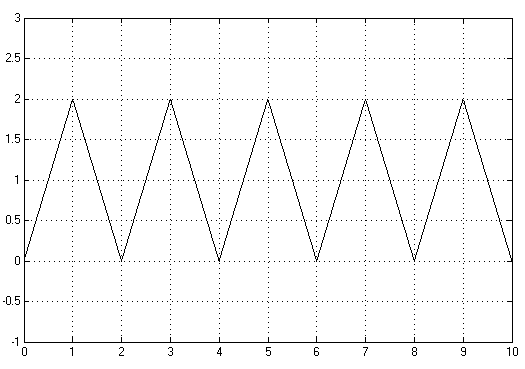• `Discrete` — Each linear segment in the repeating sequence is defined by its duration and start value. The end value of a segment is the same as its start value.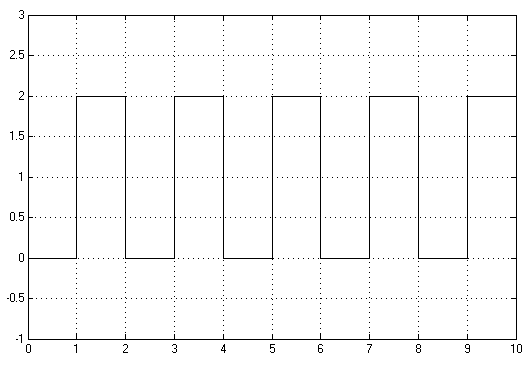Use this block to generate various types of physical signals, such as pulse, sawtooth, stair, and so on.

### Discontinuous Repeating Sequence

This example shows the mapping between the block parameter values and the resulting output signal.

Set the block parameters as shown: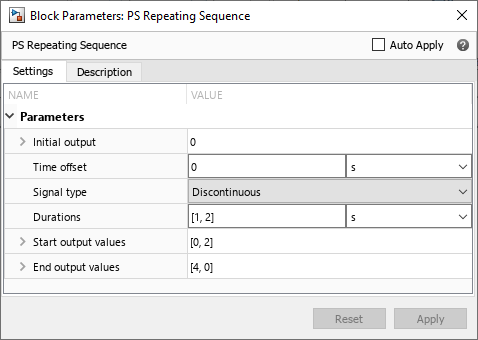The following plot shows the resulting block output.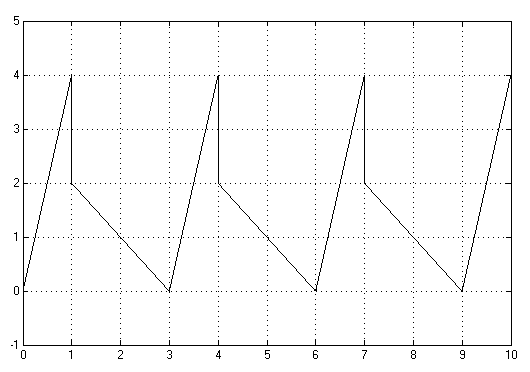The signal starts at 0 and consists of two linear segments. The duration of the first segment is 1 second, the segment starts at 0 and ends at 4. The signal is discontinuous, and the end value of the first segment is different than the start value of the second segment, therefore they are connected by a vertical line. The second segment starts at 2, lasts for 2 seconds, and ends at 0, after which the sequence repeats.

## Ports

### Output

expand all

Output physical signal.

## Parameters

expand all

The value of the output signal at time zero. The output of the block remains at this value until the simulation time reaches the Time offset value.

The value of the initial time offset, before the start of the repeating sequence. During this time, the output of the block remains at the Initial output value.

Select one of the following signal types:

• `Discontinuous` — For each linear segment in the repeating sequence, define its duration, start value, and end value. If the end value of a segment is not the same as the start value of the next segment, they are connected by a vertical line. This is the default method.

• `Continuous` — For each linear segment in the repeating sequence, define its duration and start value. The end value of a segment is the same as the start value of the next segment.

• `Discrete` — For each linear segment in the repeating sequence, define its duration and start value. The end value of a segment is the same as its start value.

Specify the linear segment durations as a 1–by–`n` row vector, where `n` is the number of linear segments in the repeating sequence. `n` must be no greater than 100. The default value specifies two linear segments, each lasting 1 second.

Specify the start values of the output signal for each linear segment as a 1–by–`n` row vector, where `n` is the number of linear segments in the repeating sequence. The size of the vector must match the size of the Durations row vector. The default value specifies that the first of the two linear segments starts at 0, and the second one starts at 2.

Specify the end values of the output signal for each linear segment as a 1–by–`n` row vector, where `n` is the number of linear segments in the repeating sequence. The size of the vector must match the size of the Durations row vector.

#### Dependencies

Enabled only when the Signal type parameter is set to `Discontinuous`. For other signal types, the end value of a segment is defined either by the start value of the next segment (`Continuous`) or the start value of the same segment (`Discrete`).

## Version History

Introduced in R2012b

expand all